﻿ Practical Investigation to Improve the Heat Transfer Performance in Elliptical Fins for Different Axis Ratios by Forced ConvectionPublications are Open
Access in this journal
Article Versions
Export Article
• Normal Style
• MLA Style
• APA Style
• Chicago Style
Research Article
Open Access Peer-reviewed

### Practical Investigation to Improve the Heat Transfer Performance in Elliptical Fins for Different Axis Ratios by Forced Convection

Hazim Abed Mohammed Al-Jewaree , Dhia K. Suker
American Journal of Mechanical Engineering. 2021, 9(1), 18-23. DOI: 10.12691/ajme-9-1-3
Received January 02, 2021; Revised January 25, 2021; Accepted February 05, 2021

### Abstract

All engineering industries have proven that there is a demand to maintain heat transfer and in many engineering production processes, an increase in the rate of thermal transfer is required.. The solution lies in adding solid bodies made externally from heat-conducting materials called fins, which in turn have been the subject of very large engineering research by changing shapes, lengths, axis, thicknesses, etc., in order to raise the efficiency of performance in heat transfer to avoid industrial problems and accidents. The materials type and surface area have direct affect of the heat transfer rate depends on the types of materials used and the surface area of the fin. One of the most popular choice is the radial annular fin due to the cylindrical primary surface where the performance of the fins is a function of many parameters, namely the heat transfer coefficient, the fin efficiency and the fins’ thermal resistance. In this research work, an experimental study to investigate the effect of fin heat transfer performance characteristics elliptical fin shape at differnt at its major and minor axis ratio (a/b) with different cooling air velocities. As a results, the optimum ratio is found to be for an elliptical shape fins for forced convection.

### 1. Introduction

A careful literature review revealed that most of the work done on geormetries of the fins is theoretical, and based on thermal and coeficcients rather than geometry. Behnia et al. 1 hae theoretical analysis of the heat transfer performance of various commonly used fin geometries in the form of circle, square, rectangle, and ellipse. Results have shown that fins with rounded shapes are better than right angled shapes which is due to the air distribution around the find at low flow, where as squared shapes can generate vortecies around the edges at high speed, and reduce the efficiency. Li et al. 2 have also found that the heat displacement rate is higher with elliptical fin pins in comparison to pin fin and the resistance of the former is lower than the latter expressed in Reynolds number ranging from 1000 to 10,000.

Chapman et al. 3 performed experimental work on using parallel court fins and the fin crossed pins in a low air flow environment and compare these fins with an elliptical pin fin heat sink. A heat sink of the same volume in their experiment are used which concluded that the overall thermal resistance of the parallel plate fin is lower than the other two designs, on the other hand, the elliptical pin fins shown higher heat transfer coefficient. Ota et al. 4, 5 have practically investigated the heat transfer and its flow around oval cylinder with axes in proportions of 1:2 and 1:3. The results show that the heat transfer coefficient of the oval cylinder is higher than the heat transfer coefficient of the circular cylinder with equal circumference and that the pressure drawing coefficients for the first is less than the previous ones. Poulikakos and Bejan 6 build a theoretical framework to explain the effect of entropy on fin dimensional variation and whether there are optimum dimensions when heat transfer by forced convection. First they developed an expression for a general fin entropy generation rate and then applied it to determine the optimum dimensions for pin fins, rectangular plate fins, trapezoidal fins, and triangular fins of rectangular cross section. It does not seem that in their studies anything added to engineering industries distinguishes them from others. Jonsson and Bjorn 7 have compared experimental results to find the thermal performance of heat platters using different fin designs such as straight and pin fins with circular, quadratic and elliptical cross sections. They have evaluated the thermal performance by comparing the heat resistance of heat exchangers by decreasing pressure at a constant velocity. They recommended the use of elliptical pin fins at high speeds and round pin fins at medium range speeds. Wirtz et. al. 8 they reached experimental results on the heat transfer performance of the pin-fin fan pool assemblies used different pin -fins shape like diamond ,cylindrical, and square and found that cylindrical pin-fins give the best overall heat transfer performance.

Laor and Kalman 9 studies the effect of heat generation on the performance of longitudinal fins, spines, and ring fins of equivalent, rectangular and triangular shapes with regular and irregular temperature distributions observed, while Mokheimer and Esmail 10 locally examined the performance of ring fins for different profiles subject to a variable heat transfer coefficient.This study is developed by studay the end wall heat transfer and total pressure drop measurements for elliptical pin fin arrays performed by Uzol and Camci 11.

Nagarani and Mayilsamy have investigated circular and ring fins and found that these are one of the most widely used options for exchanging heat. They found that the rate and efficiency of heat transfer of circular and oval ring fins were analyzed for several different environmental variables. The efficiency of the elliptical fin is more than the ring fin, if there is a restriction of space in a certain direction for the length while the vertical direction is relatively unconstrained, in practice the elliptical fin is a good choice 12.

Practical result by Al-Jawaree 13 proved that the surface temperature of elliptical fin decreased with increase in fin length along major axis. Three fins shape, namely elliptical, circular and diamond were used to to investigate the heat transfer performance at natural convection and it is fond the elliptical fin shape had more heat transfer performance than other shape used 14. In addition, extended to this work, the influence of elliptical fin axis ratio to the fin heat transfer performance was examined at free convection 15.

### 2. Experimental Methods

In this work, natural and force convection heat transfer of elliptic annular fins with different length depth ratios are experimentally investigated. Eight specimens sets with elliptical annular fin and cross section with different axis aspect ratios (a/b) are used. The following ratios are used: 2.0, 2.21, 2.44, 2.65, 2.88, 3.13, 3.38 and 3.65. Allthe speciments are of the surface area and thickness as shown in Figure 1. For examine the force convection, it is decided to use the air flow velocity from 0.15 to 0.28 m/s.

• Figure 1. Elliptical fin shape at different axis used in the experimental work for natural convection

The materials used in this experimental work is AA6061 aluminium alloy due to its good thermal conductivity, low price and low density. The material physical parameters are: thermo-physical properties = 2719 kg/m3, Cp = 871 j/kg K, K = 233 w/m K. The specifications of elliptical fin and the experimelal rig are listed in Table 1 and shown in Figure 2.

• Figure 2. The experimental rig used for natural convection

The following assumptions are made during the experimental work: the fins are of homogenious materials. The temperature around the finds are uniform, the fins’ thickness is small in comparison to the dimension, hence the temperature gradient allows fo rht eedige of the fin to be neglected. The base tube’s temperature is uniform, and no heat transfet occurs during the experiment.

### 3. Results and Discussions

The heat transfer coefficient is n of the important factor in this work, the teat transfet form the fin to the sorroundng atmosphere is affected by the air velocity and the aspect ration of the fins. The results in Table 2 show that set No. 4 (a/b = 2.65) has a higher value of heat transfer coefficient to the temperatures used about 162 w/m2 K by free convection 15, hence the axis aspect ratio of elliptical fin was fixed for practical examination the effect of fin thermal performance of forced convection as summarised in Table 2. In addtion, the table shows the heat transfer coefficient result with change of fin aspect ratio at constant air fan velocity (V = 0.37 m/s) is summarised.

• Figure 3. Cofficient of heat transfer at different elliptical fin aspect ratios

The heat transfer coefficient has a high values at elliptical fin aspect ratio (a/b) between 2 to 2.65, this will lead possibility to found the optimum axis ratio in this range. The heat transfer coefficient has range from 160 to 200 w\m2 K for this research work.

• Figure 4. Coefficient of Heat transfer at different air fan velocities

It can be deduce from the above figure the heighst value of heat transfer coefficient at 0.37 m/s fan air speed due to a good temperature distribution and heat transfer rate from the fins surface.

The fin heat transfer rate (Qfin) is calculated for different fin aspect ratios and air fan velocities at constant temperatures (100°C) and cross section area. The fin heat transfer rate can be estimated using equation 1 15.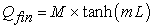(1)

where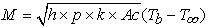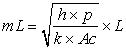(2)

where p is the fin perimeter, k is the thermal conductivity, Ac is the cross sectional area of the fin, and L is the length of the fin.

The fin heat transfer performance is measured by estimating the fin effectiveness (Ef), te fin efficiency (ηf) and overall fin thermal resistance (Ro). Hence, it has been decided to separately calculate each individual parameter. The heat transfer coefficient (h, w\m2 K) can be estimated from equations 3 and 4 16, 17.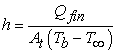(3)

where Qfin is the heat transfer from the fin surface at Ts, At is the total fin surface area, and T is the ambient temperatures.

The fin efficiency (ηfin) is defined as: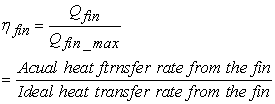(4)

This relationship enables us to determine the heat transfer from a fin when its efficiency is known. But the overall fins efficiency (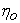) is expressed by equation 5.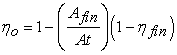(5)

The performance of fins expressed in term of the fin effectiveness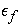is defined as: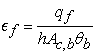(6)

where Ac,b is the surface area of fins array on the base (m2), θb is the temperature difference (Tb - Ts) and Tb is the temperature of heated surface.

The fin thermal resistance (Rfin) expressed in units of (°C/W) is defined as temperature rise per unit of power. Based on the device dissipation, the total thermal resistance can be calculated as expressed by equation 7 18, 19.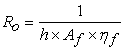(7)

where Af is the fin surface area (m2), and ηf is the fin efficiency. Equation 7 is used to calulate the thermal resistance of the fins array. A small fraction of thermal resistance in an indication to a small temperature drop across the heat sink. Hence, fins with high thermal efficiency accounts of heat flow paths for conduction-convection in the fins and convection from the prime surface. Hence, in order to study the effect of the elliptical fin axis ratio by force convection to the fin heat transfer performance, the above three parameters were calculated at the wide range of elliptical fin axis ratio using equation 7.

Actually, the experimental works divided in two parts, the first part examination of air fan velocities to the constant fin axis ratio (a/b = 2.65) and the results summarized in Table 3. But the second part of this research work done at a constant fan air velocity (V = 0.37 m\s)with a wide range of elliptical fin axis ratio , this is because an average velocity found at part one of this research work and the results are summarized in Table 4. The experiments were done at constant temperature in air surroundings excluding air currents at constant surface area for the elliptical fin (0.0395 m2) for all sets used. Hence, in order to achieve the above point elliptical fin axis ratio fixed at (a/b = 2.65) with a wide range of fan air velocities, this is because an optimum axis ratio found at free convection work. Free convection experimental results done at an air velocity equal to zero measuring the enhance of fin heat transfer performance by using the force convection. The results of the part one are illustrated in Table 3.

The fin heat transfer performance of elliptical fin at force convection is illustrated in Figure 5, Figure 6, and Figure 7. The fin effectivness increase at air fan velocity equal 0.2 m/s and then slightly decrease with increase the air velocity due to lower thermal distribution zone area on the fin surface happen at high velocities.

• Figure 5. Elliptical fins effectiveness (ε) with air fan velocities

It can be deduced from Figure 5 that all the values of fins effectiveness are more than one. This means an enhance of heat transfer rate from the fins surface for all sets used in this work. The range of fin effectiveness is from 3.2 to 3.85. The enhance the percentage by used the force convection instead the free convection can be calculated using equation 8.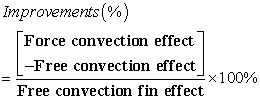(8)

This increment reaches to 177%, while the enhance percentage of overall fin efficiency raise of 80% for force convection than used for free convection at fan air velocity is equal to zero as shown in Figure 6 and Figure 7.

• Figure 6. Elliptical fin efficiency (η) with air fan velocities
• Figure 7. Overall Elliptical fin efficiency (ηo) with air fan velocities
• Figure 8. Elliptical overall thermal resistance (Ro) with air fan velocities

The results of overall efficiency conforms the fin efficiency for all air fan velocity used. At 0.2 m/s air velocity a high value of fins efficiency and lower overall thermall resistance , this is mean a high fin heat transfer performance found, with the constant elliptical fin axis ratio of a/b = 2.65.

To examine the effects of elliptical fin axis ratios to the fin heat transfer performance, the experimental work done at a constant fan velocity 0.37 m/s, temperature 100°C, 8 fis with surface area of 0.0396 m2 and wide range of elliptical fin aspect ratios from 1.5 to 3.5. The results of this part are summarised in Table 4 and illustrated in Figure 9 - Figure 12.

• Figure 9. Overall thermal resistance with different elliptical fin aspect ratios
• Figure 10. Fins effectiveness (ε) with different elliptical fin aspect ratios

It can be deduced from the above figure; all the values of fin effectiveness are more than one. So, this means an enhance of heat transfer rate from the fins surface happen for all axis ratio used in this work. The range of fin effectiveness is from 3.2 to 3.85. The fin efficiency has the range from 55 % to 75 %, but the overall fin efficiency range from 90 to 96 % due excellent design operation heat transfer fins system.

• Figure 11. Fin efficiency with different elliptical fin axis ratio
• Figure 12. Overall fin efficiency with different elliptical fin axis ratio

It can be deduce from Figure 9, Figure 10, Figure 11 & Figure 12 that set No. 4 (a/b = 2.21) has the highest efficiency and effectiveness and the least forced convection thermal resistance. While set No. 1 (a/b = 1.5) has a lowest value of heat transfer due to a large thermal distraction in the fin surface. The fins aspect ratios are very effects the fin heat transfer performance.

### 4. Conclusion

The results show that the elliptical annular fin with many axis ratios are effect the heat transfer performance of fin by both natural and force convection. The experimental results were fitted to deduce empirical correlations for force convection test. It is also found an optimum aspect ratio of 2.65 of the elliptical fin at has a high performance of heat transfer by free convection. So ,this aixis ratio fixed at practical studying the variation of air fan velocities. Considering the above points, it is concluded that the heat transfer rate of elliptical fins is better with respect to the heat transfer coefficient, shaped tube efficiency, thermal resistance, overall efficiency and effectiveness when the range of different major and manor axis ratio between 2 to 2.65. The overall fin efficiency range is from 90 to 96 % and the fin effectiveness more than one with the major and minor axis ratios and expect an increase with increasing the number of fins. While the enhance of fin heat transfer performance has a range from 80 to 180 % for this research work.

### References

  Behnia, M., Copeland, D., and Soodphadakee, D., “A Comparison of Heat Sink Geometries for Laminar Forced Convection,” Proceedings of The Sixth Intersociety Conference on Thermal and Thermo Mechanical Phenomena in Electronic Systems, Seattle, Washington, USA, May 27-30, pp. 310-315, 1998. In article  Li, Q., Chen, Z., Flechtner, U., and Warnecke, H. J.,, “Heat Transfer and Pressure Drop Characteristics in Rectangular Channels With Elliptical Pin Fins,” Int. J. Heat Fluid Flow, 19, pp. 245-250, 1998. In article View Article  Chapman, C. L., Lee, S., and Schmidt, B. L., “Thermal Performance of an Elliptical Pin Fin Heat Sink,” Proceedings of the Tenth IEEE Semi-Therm Symposium, pp. 24-31, 1994. In article  Ota, T., Aiba, S., Tsuruta, T., and Kaga, M., “Forced Convection Heat Transfer From an Elliptical Cylinder,” Bull. JSME, 26(212), pp. 262-267, 1983. In article View Article  Ota, T., Nishiyama, H., and Taoka, Y., “Heat Transfer and Flow Around an Elliptical Cylinder,” Int. J. Heat Mass Transfer, 27(10), pp. 1771-1779, 1984. In article View Article  Poulikakos, A., and Bejan, A., “Fin Geometry for Minimum Entropy Generation in Forced Convection,” J. Heat Transfer, 104, pp. 616-623, 1982. In article View Article  Jonsson, H., and Bjorn, P., “Experimental Comparison of Different Heat Sink Designs for Cooling of Electronics,” ASME J. Heat Transfer, 329(7), pp. 27-34, 1996. In article  Wirtz, R. A., Sohal, R., and Wang, H., “Thermal Performance of Pin-Fin Fan-Sink Assemblies,” J. Electron. Packag., 119, pp. 26-31, 1997. In article View Article  Laor, K., and Kalman, H., “Performance and Temperature Distributions in Different Fins With Uniform and No uniform Heat Generation,” Inst. Chem. Eng. Symp. Ser., 1(129), pp. 335-342, 1992. In article  Mokheimer, E., M. A., “Performance of Annular Fins With Different Profiles Subject to Variable Heat Transfer Coefficient,” Int. J. Heat Mass Transfer, 45(17), pp. 3631-3642, 2002. In article View Article  Uzol, O., Camci, C., “Elliptical Pin Fins as an Alternative to Circular Pin Fins for Gas Turbine Blade Cooling Applications Part 1: End wall Heat Transfer and Total Pressure Loss Characteristics,” The 46th ASME International Gas Turbine, Aero engine Congress and Exposition and Users Symposium, New Orleans, LA, 2001. In article View Article  Nagarani, N., “Mechanical Engineering Department, Anna University of Technology”, Coimbatore, K.S.R College of the Technology, Tiruchengode- 637215,Tamilnadu,India, 2010. In article  Nigerian, N., et. al., International Journal of Engineering Science and Technology. 2(7), pp. 2839-2845, 2012. In article  Al-Jewaree, H. M., “Experimentally Investigate the Heat Transfer Performance of Annular Fins,” International Journal of Scientific Engineering and Technology (IJSET), 4(10), pp. 545-548, 2015. In article View Article  Al-Jewaree, H. M., “Practically investigatr the influence of elliptical heat transfer performance at different axis ratio by free convection”. AlKitab Scientific Journal, 4, pp. 85-94, 2020. In article  Holman, F. P. and DeWitt, D. P., “Fundamentals of Heat and Mass Transfer, 4th Ed Willey, 1996. In article  Holman, J. P., “Experimental methods for engineers,” 6th Ed, McGraw-Hill, USA, 1994. In article  Incropera F.P., and DeWilh, D. P., “Fundamentals of Heat and Mass Transfer,” 14th Ed, 2011. In article  Incropera, F., DeWitt, D., “Introduction to Heat Transfer,” 4th Ed., Wiley, New York, 2002. In article  Cengel, Y. A., “Heat Transfer: A Practical Approach,” SI Units, 2nd Ed. 2004. In article

Published with license by Science and Education Publishing, Copyright © 2021 Hazim Abed Mohammed Al-Jewaree and Dhia K. SukerThis work is licensed under a Creative Commons Attribution 4.0 International License. To view a copy of this license, visit http://creativecommons.org/licenses/by/4.0/